## Bound your integral by its base times its height

### Quick description

This is an utterly trivial bound: one can bound the magnitude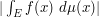of an integral by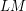, where the "base"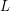is an upper bound for the measure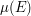of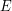, and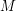is an upper bound for the magnitude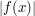of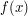on. Thus for instance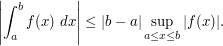If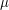is a signed or complex measure, one must ensure thatbounds the total variation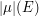and not just. (Mistakes have been made because this issue was neglected!)

One can also get lower bounds this way: ifis unsigned andis bounded from below by, andis bounded from below by, then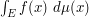is bounded from below by. Note however that it is not enough to havebounded from below byto draw a non-trivial conclusion; one must lower bounditself.

### Prerequisites

Basic measure theory; calculus; complex analysis

### Example 1

Suppose one wants to show that the contour integral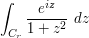goes to zero as, where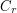is the upper semicircle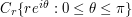. We can use "base times height" here. For the "base", observe that the length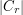of(which is also the measure ofwith respect to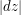) is just. For the "height", observe that on the upper semicircle,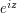has magnitude at most, while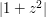is bounded from below by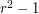(for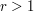), and so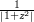is bounded from above by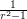, thus leading to a total height bound of. This leads to a total bound for the magnitude of integral of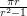, which goes to zero as, and the claim follows from the squeeze test.

(Note that this technique does not work if the denominator was linear instead of quadratic. To see how to deal with that case, see the article "bound by a Riemann sum".)

### General discussion

The base times height bound is reasonably efficient as long as

•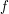does not oscillate significantly (i.e. there is no significant opportunity for cancellation); and

• the magnitude ofis reasonably uniformly distributed across(no "spikes" or large "empty regions").

When the latter condition fails, then it is often advantageous to first subdivide the integral into regions (i.e. divide and conquer) where the magnitude of the integrand is reasonably uniform, so that this method can be applied. This leads to such methods as "bound by a Riemann sum" and "dyadic decomposition".

The base times height method can also be used as a heuristic to guess the right size of an integral. If a function f is "expected" to "concentrate" on a subset of measure about, and is also believed to typically have a value of abouton this subset, then it is reasonable to expect that the final integral is of order(unless one expects lots of cancellation in the integral, in which case it could have a considerably smaller magnitude). For instance, consider the integral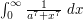for some parameter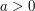. Graphing this function, we see that it attains a "height" of about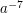and is concentrated on a "base" interval of length about, so a reasonable first guess for this integral is that it should be about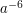, which is indeed the case (see the article "bound the integrand by something simpler" for more discussion).

The following variant of the base times height bound is also useful: if a functionhas height at mostalmost everywhere on a setof measure at most, then the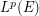offor any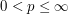is at most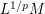.